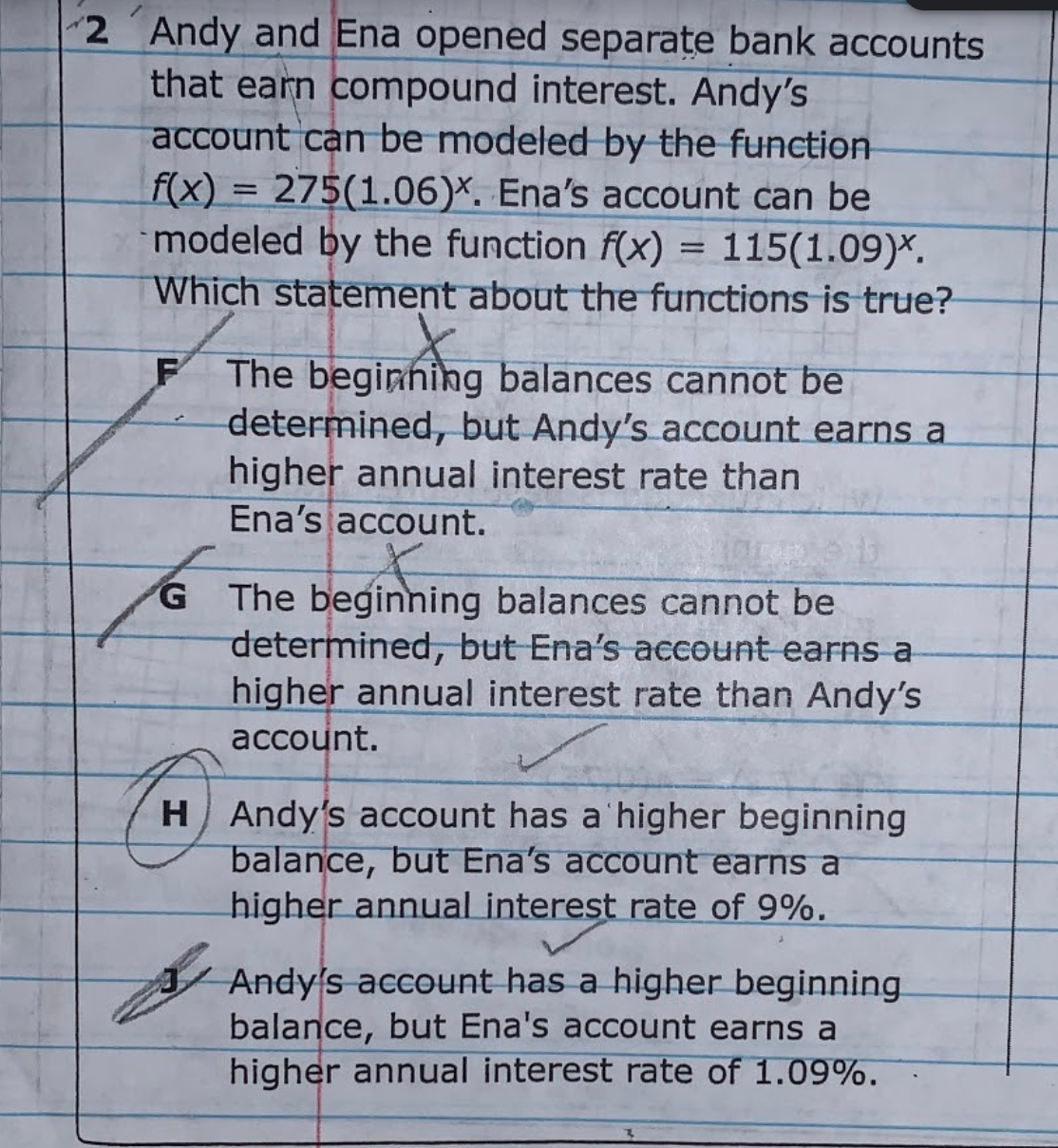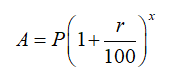# 2 Andy and Ena opened separate bank accountsthat earn compound interest. Andy'saccount can be modeled by the functionf(x) = 275(1.06)*. Ena's account can bemodeled by the function f(x) = 115(1.09)*.Which statement about the functions is true?The beginhing balances cannot bedetermined, but Andy's account earns ahigher annual interest rate thanEna's account.The beginning balances cannot bedetermined, but Ena's account earns ahigher annual interest rate than Andy'saccount.G.HAndy's account has a'higher beginningbalance, but Ena's account earns ahigher annual interest rate of 9%.A Andy's account has a higher beginning.balance, but Ena's account earns ahigher annual interest rate of 1.09%.

Question
1 views

I would like assistance on my algebra homework.help_outlineImage Transcriptionclose2 Andy and Ena opened separate bank accounts that earn compound interest. Andy's account can be modeled by the function f(x) = 275(1.06)*. Ena's account can be modeled by the function f(x) = 115(1.09)*. Which statement about the functions is true? The beginhing balances cannot be determined, but Andy's account earns a higher annual interest rate than Ena's account. The beginning balances cannot be determined, but Ena's account earns a higher annual interest rate than Andy's account. G. HAndy's account has a'higher beginning balance, but Ena's account earns a higher annual interest rate of 9%. A Andy's account has a higher beginning. balance, but Ena's account earns a higher annual interest rate of 1.09%. fullscreen
check_circle

Step 1

The amount(A) in the account after x years can be calculated as below, where P is the principal and interest rate is r% and the time of deposit is x....

### Want to see the full answer?

See Solution

#### Want to see this answer and more?

Solutions are written by subject experts who are available 24/7. Questions are typically answered within 1 hour.*

See Solution
*Response times may vary by subject and question.
Tagged in

### Algebra